## A 9.0-kg box of oranges slides from rest down a frictionless incline from a height of 5.0 m. A constant frictional force, introduced at poin

Question

A 9.0-kg box of oranges slides from rest down a frictionless incline from a height of 5.0 m. A constant frictional force, introduced at point A, brings the block to rest at point B, 19 m to the right of point A.
What is the coefficient of kinetic friction, k , of the surface from A to B?

in progress 0
2 months 2021-07-29T13:31:13+00:00 1 Answers 20 views 0

The coefficient of kinetic friction is 0.26

Explanation:

Given:

Mass of box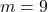kg

Initial height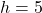m

Final height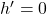m

Distance travel by block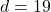m

For finding coefficient of kinetic friction,

We use conservation laws,

Work done by frictional force is equal to change in energy,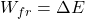Here only potential energy change so we can write,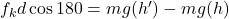Here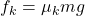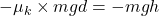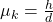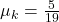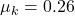Therefore, the coefficient of kinetic friction is 0.26